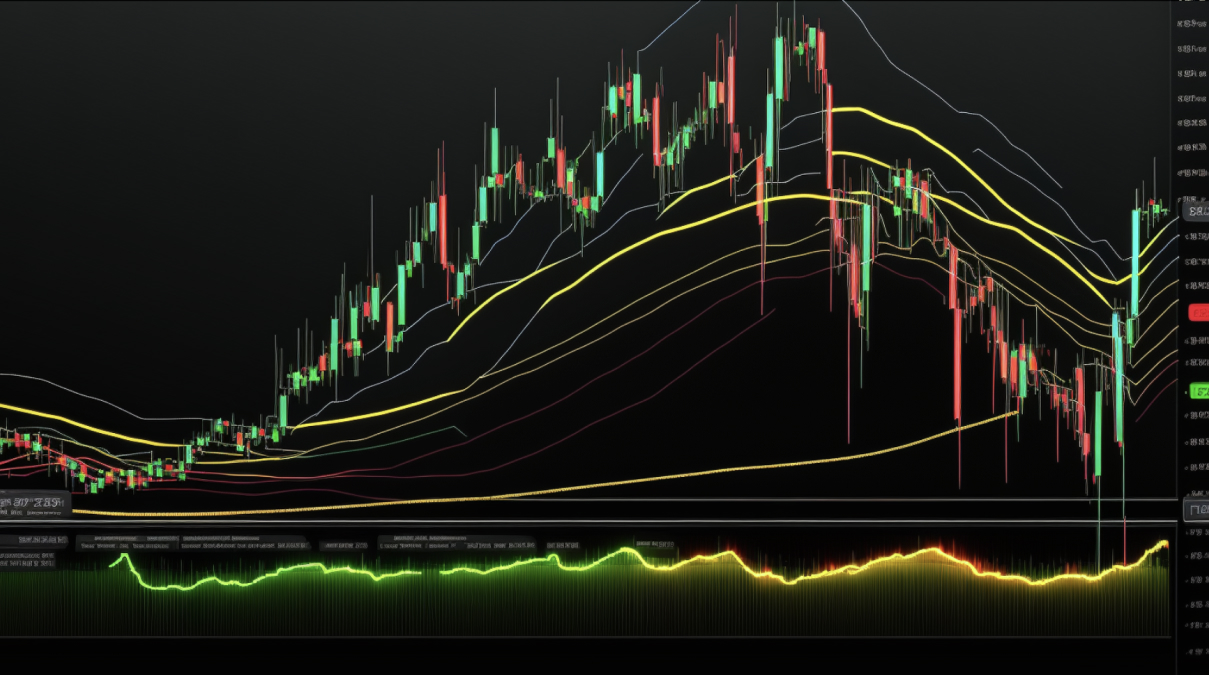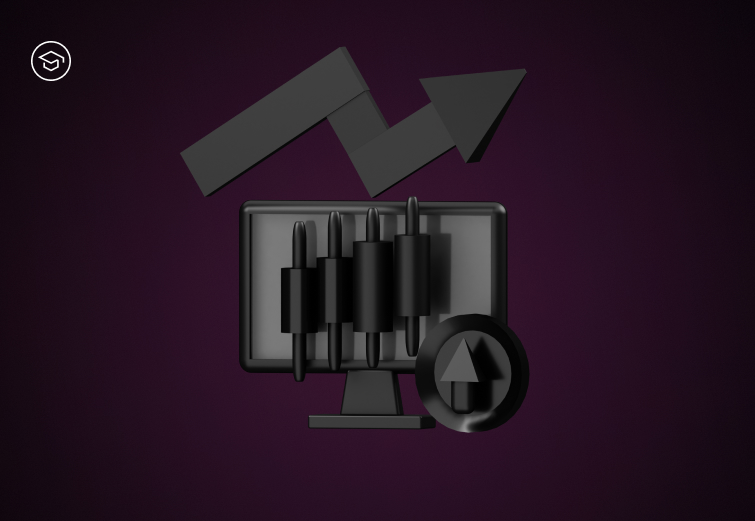STC Educational Panel

## Exponential Moving Average (EMA) and Simple Moving Average (SMA)

March 24, 2023
11mAn Exponential Moving Average (EMA) is a fundamental technical indicator used in Technical Analysis. This weighted moving average places significance on the most recent data to forecast future price direction.

EMA tracks an asset's price over selected time frames like 10, 50, or 200 days. Investors typically calculate 12-day and 26-day EMA for short-term averages and opt for 50- and 200-day to spot long-term trends.

As it concentrates on the most recent records, EMA can be more sensitive to price changes. This makes it relatively widespread among day traders.

#### How to use Exponential Moving Averages (EMA) to determine market trends and reversals?‍

EMA is typically used to determine trends and reversals. Reversals occur when an upward trend starts to go down, or a downtrend starts to go up. To spot a reversal, you can analyze both short-term and long-term EMA and see how they correlate.

As an example, if your 26-day and 50-day EMA indicators have a crossover, it may indicate a trend reversal.

If the price of your asset remains above the EMA, the upward trend will likely continue. A market reversal can be indicated as soon as the price drops below the moving average.

You can use EMA to determine support and resistance levels, as they are dynamic and based on recent price action, just like EMA.

#### How to calculate EMA?

The calculation of EMA involves three steps: calculating the SMA for a specific period, then the weighting multiplier and applying the EMA formula.

Calculate the SMA – for example, if you are calculating the 10-day EMA, you need to calculate the SMA for 10 days (which comes down to adding up the closing prices of the last 10 days and dividing the total by 10).

Calculate the weighting multiplier, based on the formula:

[2 ÷ (selected time period + 1)]

For a 10-day EMA, it would be: [2/(10+1)] = 0.1818.

Lastly, apply the EMA formula:

EMA = Price(t) x 2/(N+1) + EMA(y) x (1-k),
where
t = today
y = yesterday
N - number of days (in our example, 10)

One of the most significant benefits of using EMA is that it can detect short-term volatility moves faster than many other technical indicators. EMA is also known for its quick response to price fluctuations.

On the other hand, it may provide false signals when it is too early to determine the overall trading situation.

#### Simple Moving Average (SMA)‍

Simple Moving Average (SMA) is the arithmetic average of the closing prices over a specified period, such as 10 days, 20 days, or 50 days.

For example, if you are calculating the 10-day SMA, you would add up the closing prices of the last 10 days and divide the total by 10.

The result is the SMA for that day.

Assuming our assets’ closing prices are as follows: \$40, \$42, \$43, \$46, \$38, \$41 \$47, \$44, \$43, \$41 – SMA calculation would look like this:
\$40 + \$42 + \$43 + \$46 + \$38 + \$41+ \$47 + \$44 + \$43 + \$41 = 425

10-period SMA = \$425/10 = 42,5.

SMA is commonly used to smooth price data and technical indicators, providing a clearer view of an asset's long-term trend. Investors may use the SMA to identify potential entry and exit points.

A bullish trend may be signaled if an asset's price crosses above its SMA, while a bearish trend may be indicated by a crossing below its SMA.

One of the main advantages of the SMA is that it provides a smoothed line of price data, less prone to whipsaws in response to slight, temporary fluctuations. The SMA can also be used to identify potential support and resistance levels for assets. Moreover, it is easy to calculate and customizable to suit trading strategies and preferences.

As for disadvantages, the SMA may not provide timely signals for traders looking to quickly enter or exit. It may also generate false signals, especially in volatile markets, which can lead to losses for traders.

#### Exponential Moving Average (EMA) vs. Simple Moving Average (SMA)

The main difference between these two indicators is how they calculate the average price. The SMA calculates it over a specified period by taking the arithmetic mean of the closing prices. The EMA, on the other hand, puts more weight on recent prices, giving greater significance to the most recent data points.

While every price is “treated equally” in SMA, the most recent prices are more crucial in EMA. Consequently, SMA does not adjust to market changes as quickly as EMA.

#### Summary

The Exponential Moving Average and Simple Moving Average are two technical indicators utilized in financial analysis.

While the SMA calculates the average price over a specific time by taking the arithmetic mean of the closing prices, the EMA places more weight on recent prices. The EMA is more responsive to recent price changes than the SMA. Investors can use these indicators to identify trends and reversals, determine support and resistance levels, and make trading decisions.

However, they should be aware of the advantages and disadvantages of using these indicators in their trading practices.

Complete quiz
Exponential Moving Average (EMA) and Simple Moving Average (SMA)Intermediate

### What is token burning and why is it important?

Token burning can be beneficial for long-term investors but may negatively impact traders in the short term by reducing liquidity and causing price fluctuations.Intermediate

### Stock-to-Flow (S2F) Model

The Stock-to-Flow (S2F) model is an analytical tool used in cryptocurrencies to foresee future asset prices by calculating the ratio of the total supply to the annual incremental supply. The model is based on the assumption that a higher S2F ratio indicates higher rarity and may result in an increase in the asset's value.Intermediate